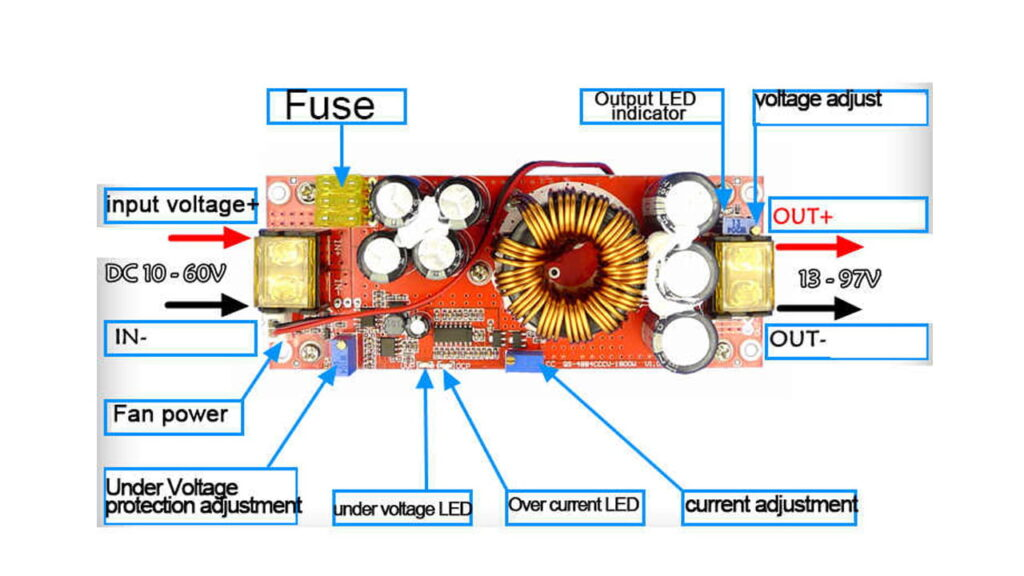# 1800 Watt Constant Voltage Boost Converter

Looking for 1800w boost converter? This boost converter has high efficiency, long service life and low power consumption. High quality boost converter with low price. You can use this boost converter for high current . Here you can find genuine review about 1800 watt Constant Voltage Boost Converter.

## 1800 watt Constant Voltage Boost Converter Specification

Input voltage: DC10-60V

Input current: 10-30V input voltage, maximum current 30A

31-60V input voltage, maximum current 25A

Static working current: 15mA (12V liters 20V, the higher the output voltage, the static current will increase)

Output voltage: 12-97V continuously adjustable

Output current: 20A MAX More than 15A, please strengthen the heat dissipation (related to the input and output voltage difference, the larger the differential pressure, the smaller the output current)

Constant current range: 0.8-20A (+/-0.3A)

Input anti-reverse connection: Yes (150A power MOS anti-reverse)

Low battery protection: self-recovery (10-45V adjustable)

Working temperature: -40~+85 degrees (enhance heat dissipation when the ambient temperature is too high)

Working frequency: 150KHz

Conversion efficiency: 92%-97% (efficiency is related to input, output voltage, current, differential pressure. Low differential pressure)

Input overcurrent protection: Yes (input over 35A, automatic protection, power does not boost)

Short circuit protection: Yes (input 40A fuse) Double short circuit protection, safer to use.

Input reverse connection protection: Yes (150A power MOS tube anti-reverse. Can be reversed for a long time)

Output power: = input voltage * 30A, such as: input 12V * 30A = 360W The maximum power when inputting 12V is 360W

Input voltage *30A For example: input 24V * 30A = 720W, that is: the maximum power is 720W when inputting 24V, Input 60V*30A = 1800W

Power description:

Since the input current is 30A at 10-30V and the input current is 25A at 31-60V, the output power is related to the input voltage. The higher the input voltage, the higher the power.

(Input voltage * input current is equal to the whole machine power) For example, the input 12V*30A power is 360W. The input 24V*30A is 720W. When the input voltage is 36V, the input current is 25A.Induction heating is the process of heating an electrically conducting object by electromagnetic induction, through heat generated in the object by eddy currents Read More…